# NCERT Solutions for Class 8 Maths Chapter 2 Linear Equations in One Variable Ex 2.5

NCERT Solutions for Class 8 Maths Chapter 2 Linear Equations in One Variable Ex 2.5 are part of NCERT Solutions for Class 8 Maths. Here we have given NCERT Solutions for Class 8 Maths Chapter 2 Linear Equations in One Variable Ex 2.5.

 Board CBSE Textbook NCERT Class Class 8 Subject Maths Chapter Chapter 2 Chapter Name Linear Equations in One Variable Exercise Ex 2.5 Number of Questions Solved 2 Category NCERT Solutions

## NCERT Solutions for Class 8 Maths Chapter 2 Linear Equations in One Variable Ex 2.5

Question 1.
Solve the following linear equations:
1. $$\frac { x }{ 2 } -\frac { 1 }{ 5 } =\frac { x }{ 3 } +\frac { 1 }{ 4 }$$
2. $$\frac { n }{ 2 } -\frac { 3n }{ 4 } +\frac { 5n }{ 6 } =21$$
3. $$x+7-\frac { 8x }{ 3 } =\frac { 17 }{ 6 } -\frac { 5x }{ 2 }$$
4. $$\frac { x-5 }{ 3 } =\frac { x-3 }{ 5 }$$
5. $$\frac { 3t-2 }{ 4 } -\frac { 2t+3 }{ 3 } =\frac { 2 }{ 3 } -t$$
6. $$m-\frac { m-1 }{ 2 } =1-\frac { m-2 }{ 3 }$$
Solution.
1. $$\frac { x }{ 2 } -\frac { 1 }{ 5 } =\frac { x }{ 3 } +\frac { 1 }{ 4 }$$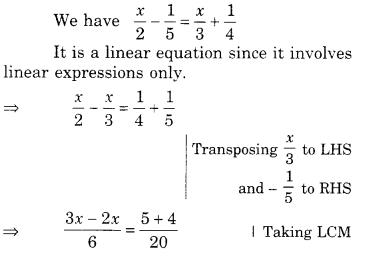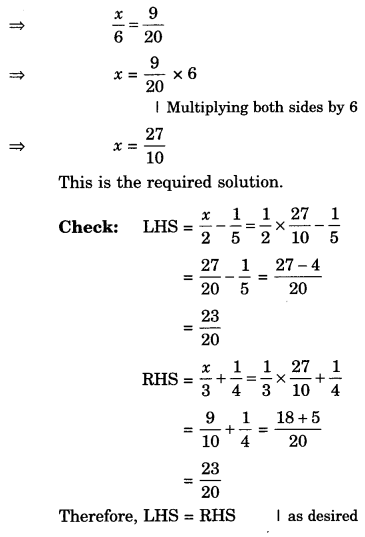2. $$\frac { n }{ 2 } -\frac { 3n }{ 4 } +\frac { 5n }{ 6 } =21$$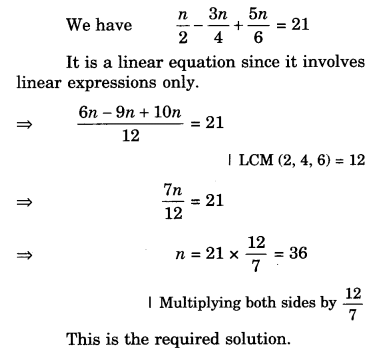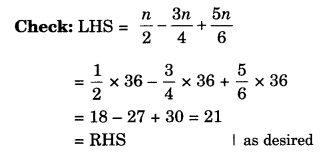3. $$x+7-\frac { 8x }{ 3 } =\frac { 17 }{ 6 } -\frac { 5x }{ 2 }$$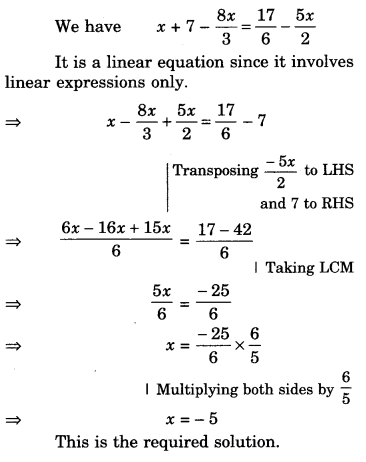4. $$\frac { x-5 }{ 3 } =\frac { x-3 }{ 5 }$$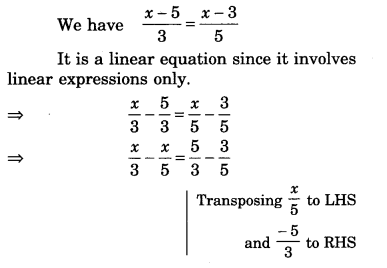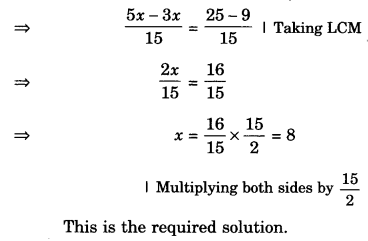5. $$\frac { 3t-2 }{ 4 } -\frac { 2t+3 }{ 3 } =\frac { 2 }{ 3 } -t$$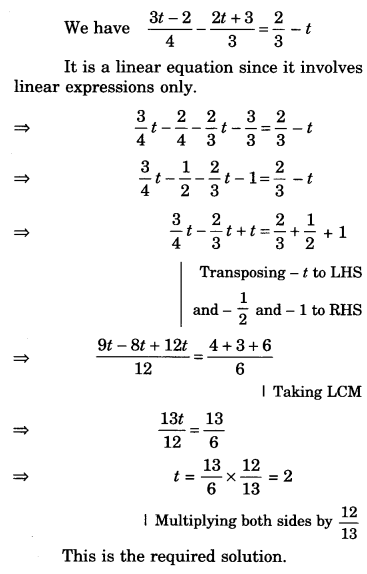6. $$m-\frac { m-1 }{ 2 } =1-\frac { m-2 }{ 3 }$$
We have $$m-\frac { m-1 }{ 2 } =1-\frac { m-2 }{ 3 }$$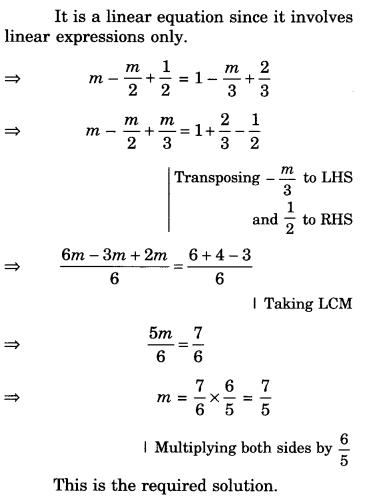Question 2.
Simplify and solve the following linear equations:
7. 3(t-3)=5(2t+1)
8. 15(y-4)-2(y-9)+5 (y+6) = 0
9. 3(5z-7)-2 (9z-11)=4 (8z-13)-17
10. 0.25(4f-3) = 0.05 (10f-9).
Solution.
7. 3(t-3)=5(2t+1)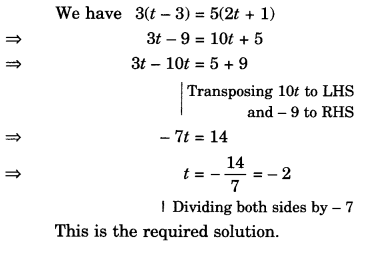8. 15(y-4)-2(y-9)+5 (y+6) = 0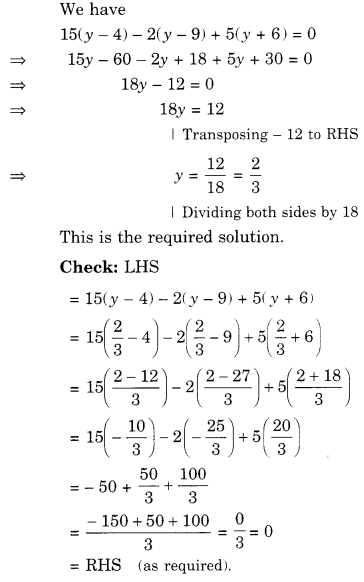9. 3(5z-7)-2 (9z-11)=4 (8z-13)-17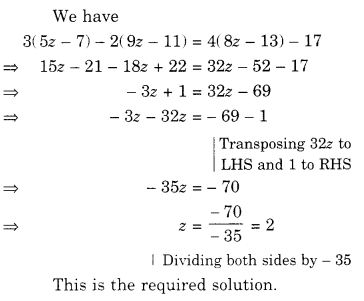10. 0.25(4f-3) = 0.05 (10f-9)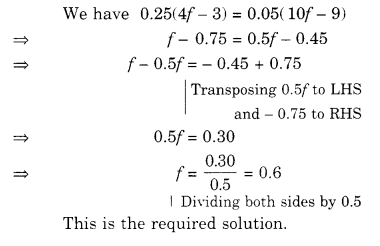We hope the NCERT Solutions for Class 8 Maths Chapter 2 Linear Equations in One Variable Ex 2.5 help you. If you have any query regarding NCERT Solutions for Class 8 Maths Chapter 2 Linear Equations in One Variable Ex 2.5, drop a comment below and we will get back to you at the earliest.